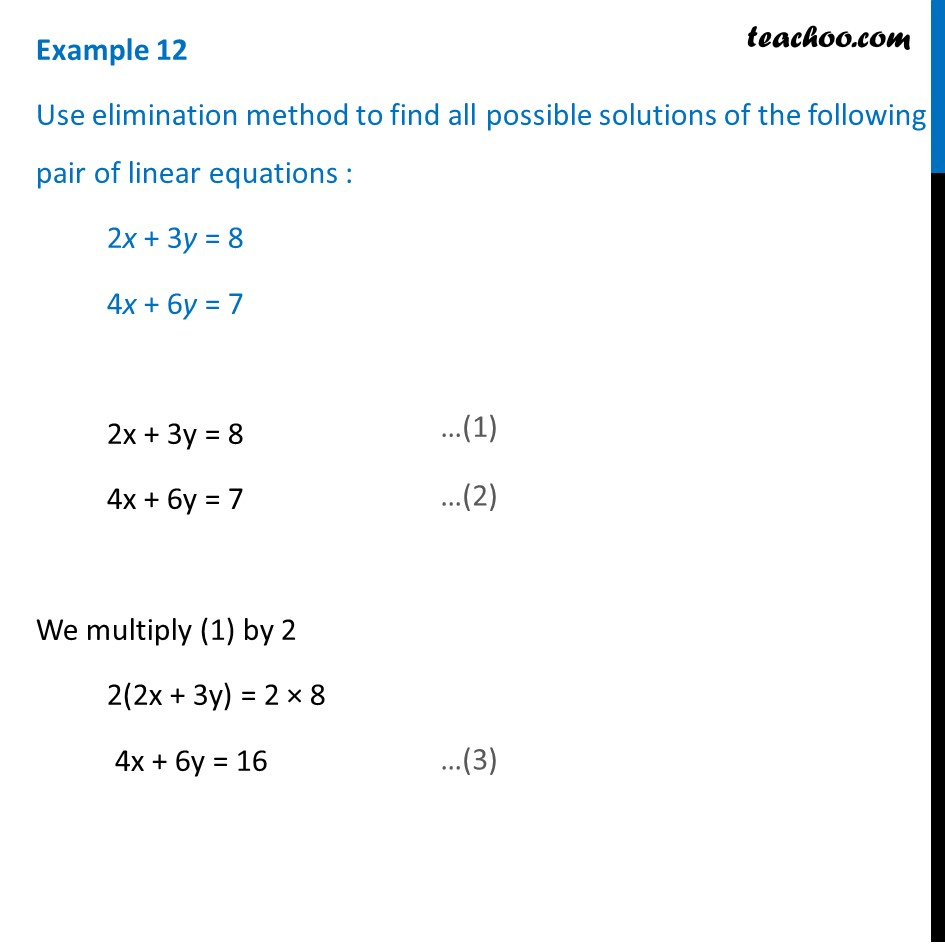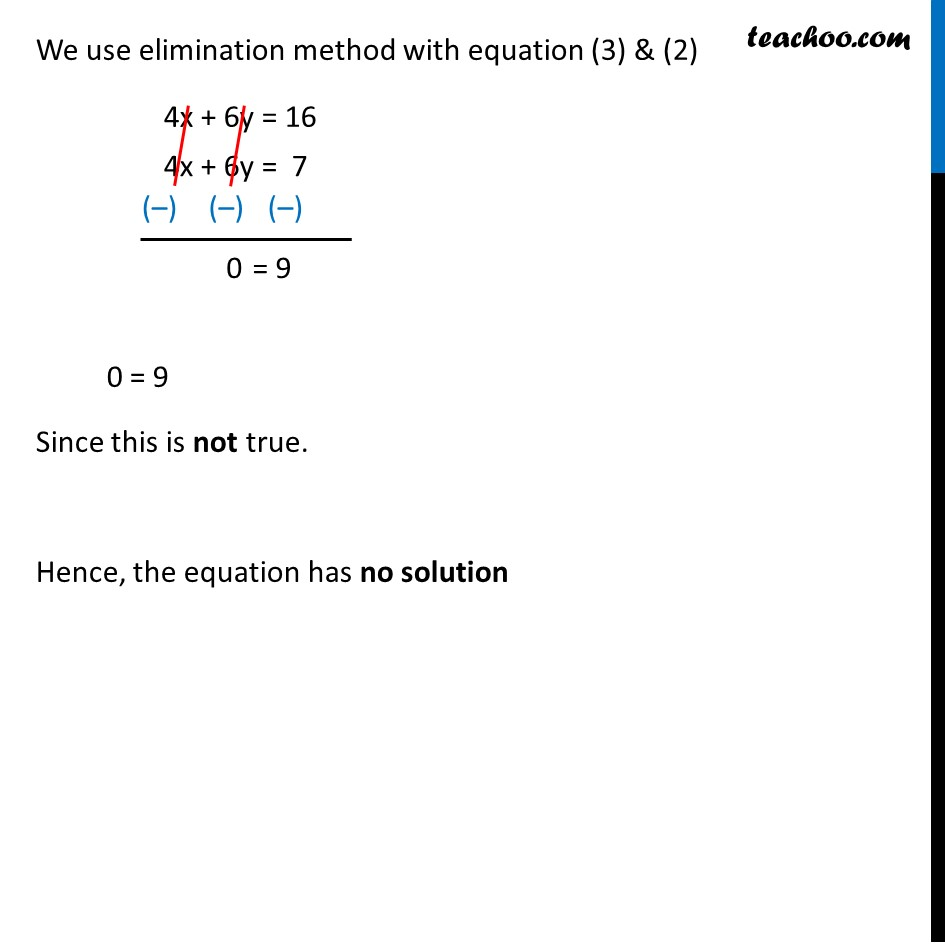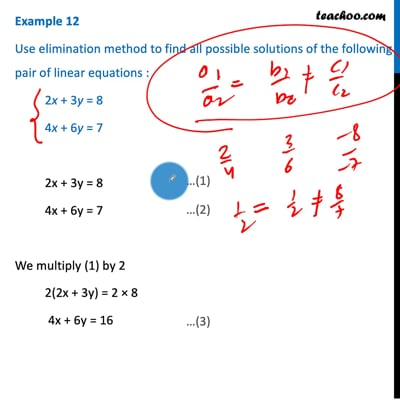Examples

Chapter 3 Class 10 Pair of Linear Equations in Two Variables (Term 1)
Serial order wiseThis video is only available for Teachoo black users

### Transcript

Example 12 Use elimination method to find all possible solutions of the following pair of linear equations : 2x + 3y = 8 4x + 6y = 7 2x + 3y = 8 4x + 6y = 7 We multiply (1) by 2 2(2x + 3y) = 2 × 8 4x + 6y = 16 We use elimination method with equation (3) & (2) 0 = 9 Since this is not true. Hence, the equation has no solution